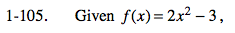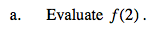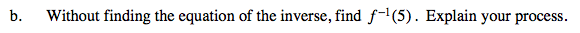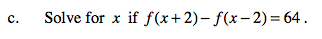### Home > CALC > Chapter 1 > Lesson 1.3.1 > Problem1-105

1-105.Substitute 2 in for every x in the equation.

2(2²) − 3

f(2) = 5Recall that that the inputs and outputs are switched for the inverse of a function.

What was the result you found in part (a)? How can you use that to find f −1(5)?f(x + 2) = 2((x + 2)²) - 3 f(x − 2) = 2((x − 2)²) - 3

Substitute these formulas into the equation above. [2((x + 2)²) − 3] − [2((x − 2)²) − 3] = 64

Solve for x.